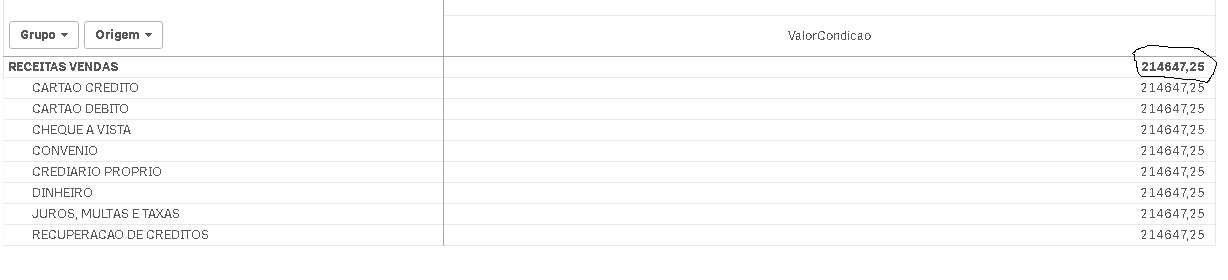# New to Qlik Sense

Discussion board where members can get started with Qlik Sense.Not applicable

## Problems with calculated dimension and hierarchy

I have the following scenario:

I have a pivot table with a group size and other subgroup and a measurement with the name value. The subgroup size has an association with the size group.

In some dimensions were made calculated fields to change the sum of the total size group. As an example only add some subgroups that are within a certain group, the pattern for summing all.

The following expression:

if (Group <> 'RECIPES' Sum (value), if (Only (Group <> 'SALES REVENUE'), Value, SUM (total {\$ <Subgroup = { 'CARD CREDIT'}>} Value) + SUM ( Total {\$ <subgroup = { 'DEBIT CARD'}>} Value)))

The problem:

The value are being replicated in all subgroups. If my goal and only change the total value of the group.

Tags (1)
4 RepliesMVP

## Re: Problems with calculated dimension and hierarchy

Would you be able to share a sample? Difficult to understand the issue without looking at itNot applicable

## Re: Problems with calculated dimension and hierarchyThe total is doubling the bass lines

HighlightedMVP

## Re: Problems with calculated dimension and hierarchy

Still not sure I understand the issue. What would you expect to see?Not applicable

## Re: Problems with calculated dimension and hierarchy

If the total is a calculated field of lines 1 and 2 that are below the group. However this total is being applied on all lines and should be applied only in the total group is the level 1 of the hierarchy.The figure below is the result that accurate, the calculated field where the group size is just the sum of the line 1 and 2 which is a dimension subgroup.

Recapping I have two two dimensions and a sublevel and the other. I am Group size and subgroup size that are children Group.

However the calculation that I am using is calculating correctly but is changing the value of all subgroup of the items below as is the image you insert in the previous post.